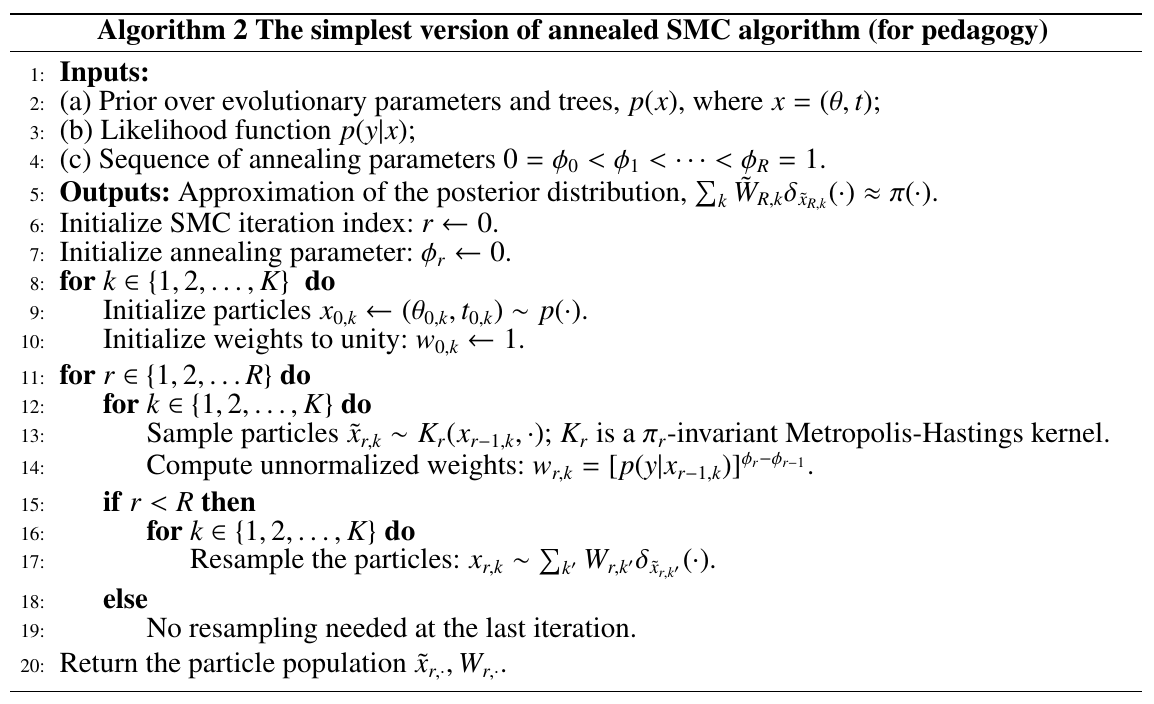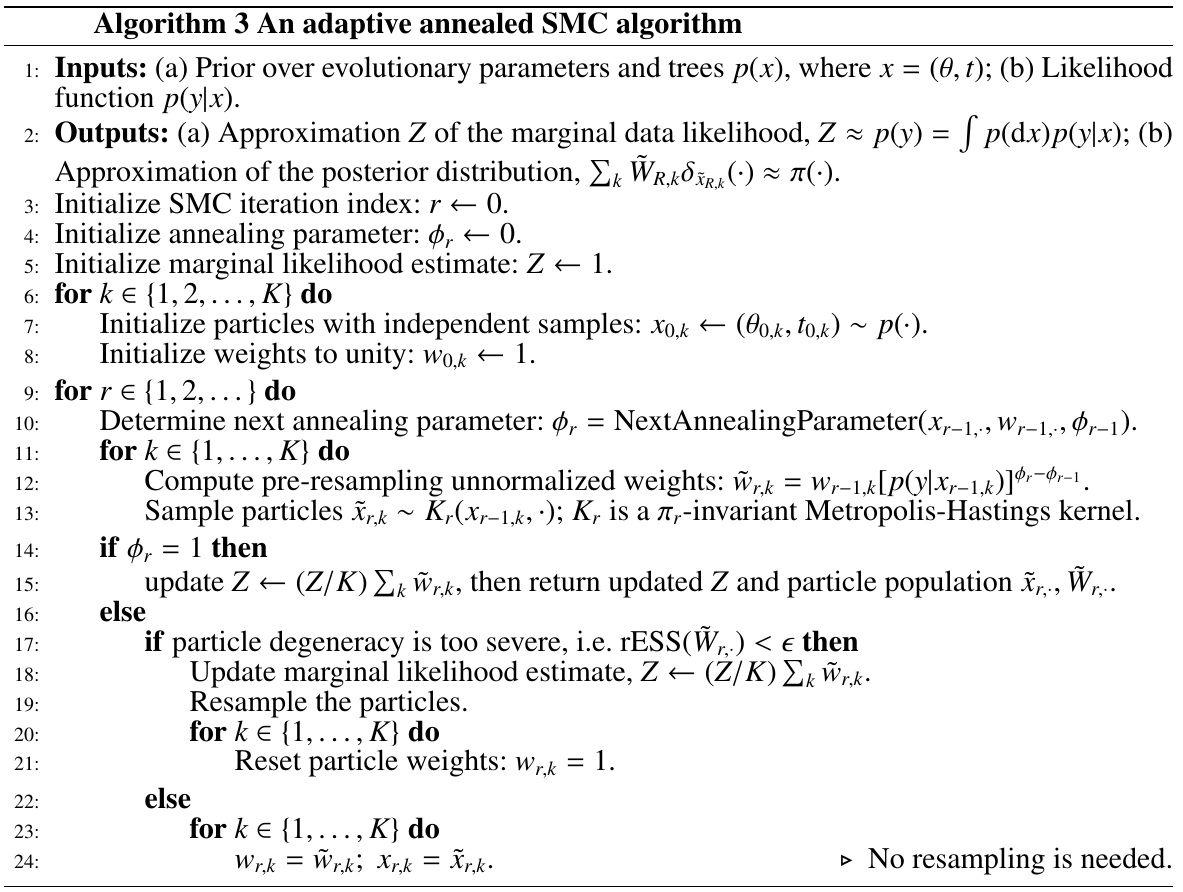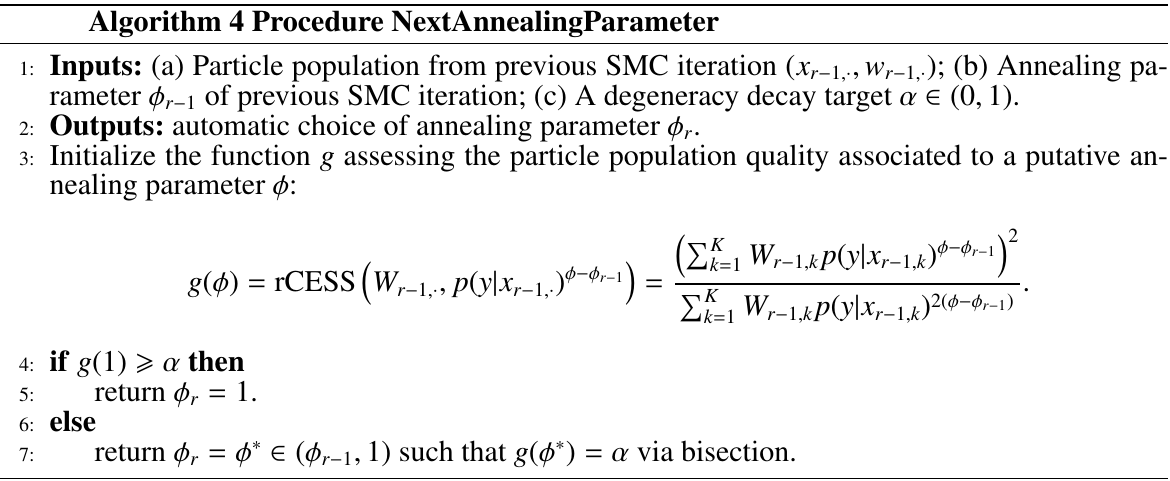# Annealed SMC for Bayesian Phylogenetics

## Abstract

### Proposal

an “embarrassingly parallel” method for Bayesian phylogenetic inference, annealed SMC, based on recent advances in the SMC literature such as adaptive determination of annealing parameters.

provides an approximate posterior distribution over trees and evolutionary parameters as well as an unbiased estimator for the marginal likelihood, which can be used to test the correctness of posterior simulation software.

evaluate the performance of phylogenetic annealed SMC by reviewing and comparing with other computational Bayesian phylogenetic methods, in particular, different marginal likelihood estimation methods.

## Introduction

The Bayesian paradigm is widely used in systematic biology, principally for the purpose of phylogenetic reconstruction as well as for evaluating the empirical support of evolutionary models.

Bayesian phylogenetic reconstruction and model selection involve an intractable sum over topologies as well as a high dimensional integral over branch lengths and evolutionary parameters. MCMC can be used to approximate posterior distributions.

Two key limitations of MCMC phylogenetic methods:

• MCMC methods do not readily take advantage of highly parallel computer architectures. While there are techniques available to parallelize phylogenetic MCMC methods, they are not “embarassingly parallel”: e.g., parallel Metropolis coupled MCMC quickly reaches a point where the addition of cores achieves diminishing returns.
• Bayes factor for model selection

Several methods to estimate marginal likelihoods on MCMC methods: have different drawbacks, see Fourment, M., Magee, A. F., Whidden, C., Bilge, A., Matsen IV, F. A., & Minin, V. N. (2018). 19 dubious ways to compute the marginal likelihood of a phylogenetic tree topology. for more details.

One limitation shared by all MCMC-based marginal likelihood estimator is that they are generally biased.

SMC methods provides a flexible framework to construct unbiased estimators and past work has shown they can be very efficient in a phylogenetic context. One drawback caused by the high degree of flexibility that comes with SMC is that the phylogenetic SMC algorithms developed so far are non-trivial to adapt to existing MCMC-based phylogenetic frameworks.

The paper propose the so-called phylogenetic annealed SMC, a different construction based on annealed importance sampling (AIS), which yields an SMC method that is in a sense much closer to standard MCMC, while providing unbiased estimators of the marginal likelihood. The proposed method can directly make use of any existing phylogenetic MCMC proposals, a rich literature covering many kinds of phylogenetic trees.

## Literature Review

A powerful feature of the general SMC framework is that the space on which the distributions $\pi_r$ are defined is allowed to vary from iteration to the next.

Several “bottom up” approaches have been proposed which allow more efficient reuse of intermediate stages of the Felsenstein pruning recursions. For these methods, the intermediate distributions are defined over forests over the observed taxa, and hence their dimensionality increases with $r$.

Someone use a sequence of targets where $\pi_r$ is a tree over the first $r$ tips. This construction is especially useful in scenarios where taxonomic data comes in an online fashion.

SMC has been used for Bayesian phylogenetic analysis based on infinite state-space evolutionary models or for joint inference of transition networks.

Someone has explored a combination of reversible jump methods with phylogenetic models.

One drawback of letting the dimensionality of $\pi_r$ vary with $r$ as all the above methods do, is that it makes it significantly harder to incorporate SMC into existing Bayesian phylogenetic inference packages. But the authors argue that in their method, the target distributions $\pi_r$ are all defined in the same space. The annealed SMC framework in this context utilizes MH kernels in the inner loop but combines them in a different fashion compared to standard MCMC algorithms, or even compared to parallel tempering MCMC algorithms.

## Setup and Notation

• $t$: a phylogenetic tree with tips labelled by a fixed set of operational taxonomic units $X$, and it encapsulates the tree topology and a set of positive branch lengths.
• $\theta$: evolutionary parameters, such as the parameters of a family of rate matrices in the general time reversible (GTR) model, or diffusion parameters in the case of continuous traits.
• $x=(t,\theta)$: latent variable
• $y$: observed data indexed by the tips $X$ of $t$.
• $p(y\mid x)$: the likelihood function, assume that for any hypothesized tree and parameters, the value $p(y\mid x)$ can be computed efficiently. This assumption is sometimes called pointwise computation, and the computation is done with some version of Felsenstein pruning.
• $p(x)$: a prior on the parameters and trees, which we assume can also be computed pointwise efficiently.
• $\gamma(x)=p(x)p(y\mid x)$: a joint distribution.
• $\pi(x)=\frac{\gamma(x)}{\int \gamma(x’)dx’}$: posterior distribution, which we want to approximate. Here the integral and $dx’$ are viewed in an abstract sense and include both summation over discrete latent variables such as topologies and standard integration over continuous spaces.
• $Z=p(y)=\int \gamma(x)dx$: the marginal likelihood under the model specified by the prior and likelihood functions. Computation of this quantity, also called the normalization constant or evidence, is the main challenge involved when doing Bayesian model selection.
• $\int \pi(x)f(x)dx$: expectations with respect to the posterior distribution, which are characterized by a real-valued function of interest $f$. For example, a posterior clade support for a subset $X’\subset X$ of the leaves $X$, $f(x)=f(t,\theta)=1[t\; \text{admits}\; X’\;\text{as a clade}]$

## Annealed SMC for Phylogenetics

### Sequences of Distributions

In standard MCMC methods, we are interested in a single probability distribution, the posterior distribution. Reasons why we may use a sequence of distributions rather than only one:

• online problem, where the data is revealed sequentially and we want to perform inference sequentially in time based on the data available so far.
• having multiple distributions is to facilitate the exploration of the state space. Achieved by raising the likelihood term to a power $\phi_r$ between zero and one, which multiply with the prior $\gamma_r(x)=p(y\mid x)^{\phi_r}p(x)$. MCMC may get stuck in a region of the space of phylogenetic trees around the initial value. This may happen around a local maximum (mode) in the posterior density. Such a region is sometimes called a “basin of attraction”, and no single basin of attraction may be enough to well represent the full posterior distribution. A small values of $\phi_r$ flattens the posterior and makes MCMC samplers move easily between the different basins of attractions.
• a “tall data” problem, e.g., biological sequences with a large number of sites. When the number of sites is large, evaluation of the unnormalized posterior $\gamma_r(x)$ is computationally expensive. The idea of data subsampling can be used to define the sequence of distributions.

## Basic Annealed SMC algorithm

At $r=1,\ldots,R$ iteration, maintain a collection indexed by $k\in\{1,2,\ldots,K\}$ of imputed latent states $x_{r,k}$, each paired with a non-negative number called a weight $w_{r,k}$; such a pair is called a particle.

The goal behind the annealed SMC algorithm is to progressively transform this prior distribution approximation into a posterior distribution approximation.

An SMC proposal distribution $K_r(x_{r-1,k},x_{r,k})$ used to sample a particle for the next iteration given a particle from the previous iteration. Since $x_{r-1,k}$ and $x_{r,k}$ have the same dimensionality in our setup, it is tempting to use MCMC proposals $q_r(x_{r-1,k},x_{r,k})$ in order to build SMC proposals, such as subtree prune and regraft moves, and Gaussian proposals for the continuous parameters and branch lengths.

Advantages of using MCMC proposals as the basis of SMC proposals.

• leverage a rich literature on the topic
• make it easier to add SMC support to existing MCMC-based software libraries
• makes certain benchmark comparison between SMC and MCMC more direct.

Naively picking the SMC proposal directly from an MCMC proposal, $K_r(x_{r-1,k},x_{r,k})=q_r(x_{r-1,k},x_{r,k})$. The magnitude of the fluctuation of the weights of the particles from one iteration to the next, $\Vert W_{r-1,\cdot}-W_{r,\cdot}\Vert$ does not converge to zero when the annealing parameter change $\phi_r-\phi_{r-1}$ goes to zero.

To avoid this, use as SMC proposal as the accept-reject Metropolis-Hastings transition probability based on $q_r$, called a Metropolized porposal.

In the annealed SMC algorithm, the weighting and proposal steps can be interchanged. This is different from standard SMC algorithms.

An estimate of the marginal likelihood $p(y)$ is given by the product of the average unnormalized weights.

$\hat Z_K = \prod_{r=1}^R\frac 1K\sum_{k=1}^Kw_{r,k}\,.$## Adaptive Mechanisms for Annealed SMC

Two schemes:

• relax the assumption that resampling is performed at every step
• automatic construction of the annealing parameter sequence

debiased adaptive annealed SMC: run the algorithm twice, a first time to adaptively determine the resampling and annealing schedules, and then a second independent time using the schedule fixed in the first pass.

Both adaptive methods rely on being able to assess the quality of a particle approximation.

Measuring particle degeneracy:

• Effective Sample Size (ESS)
• conditional ESS (CESS)
• Relative ESS (rESS)
• Relative CESS (rCESS)

Both Dynamic resampling and Adaptive determination of annealing parameters are based on relative conditional ESS, while the former need to trace back until the previous resampling step and the latter only focus on the degeneracy of a single iteration.where## Alternatives to estimate marginal likelihood

### Stepping Stone

Introduce a list of annealed posterior distributions connecting the posterior distribution and the prior distribution.

The importance sampling approximation would be poor if the two successive distributions do not have enough overlaps. Linked Importance Sampling improves the performance of importance sampling by introducing bridge distributions, such as “geometric” bridge: $\gamma_{(d-1)d}(x)=\sqrt{\gamma_{d-1}(x)\gamma(d)(x)}$.

## Simulations

1. generate random unrooted trees, including topology and branch lengths, as the reference trees.
2. simulate DNA sequences using the K2P model
3. use majority-rule consensus tree to summarize the weighted phylogenetic tree samples obtained from annealed SMC.
4. measure the distance between each estimated consensus tree its associated reference tree using three types of distance metrics:
• the normalized Robinson-Foulds metric
• the partition metric
• the Kuhner-Felsenstein metric

Published in categories Report